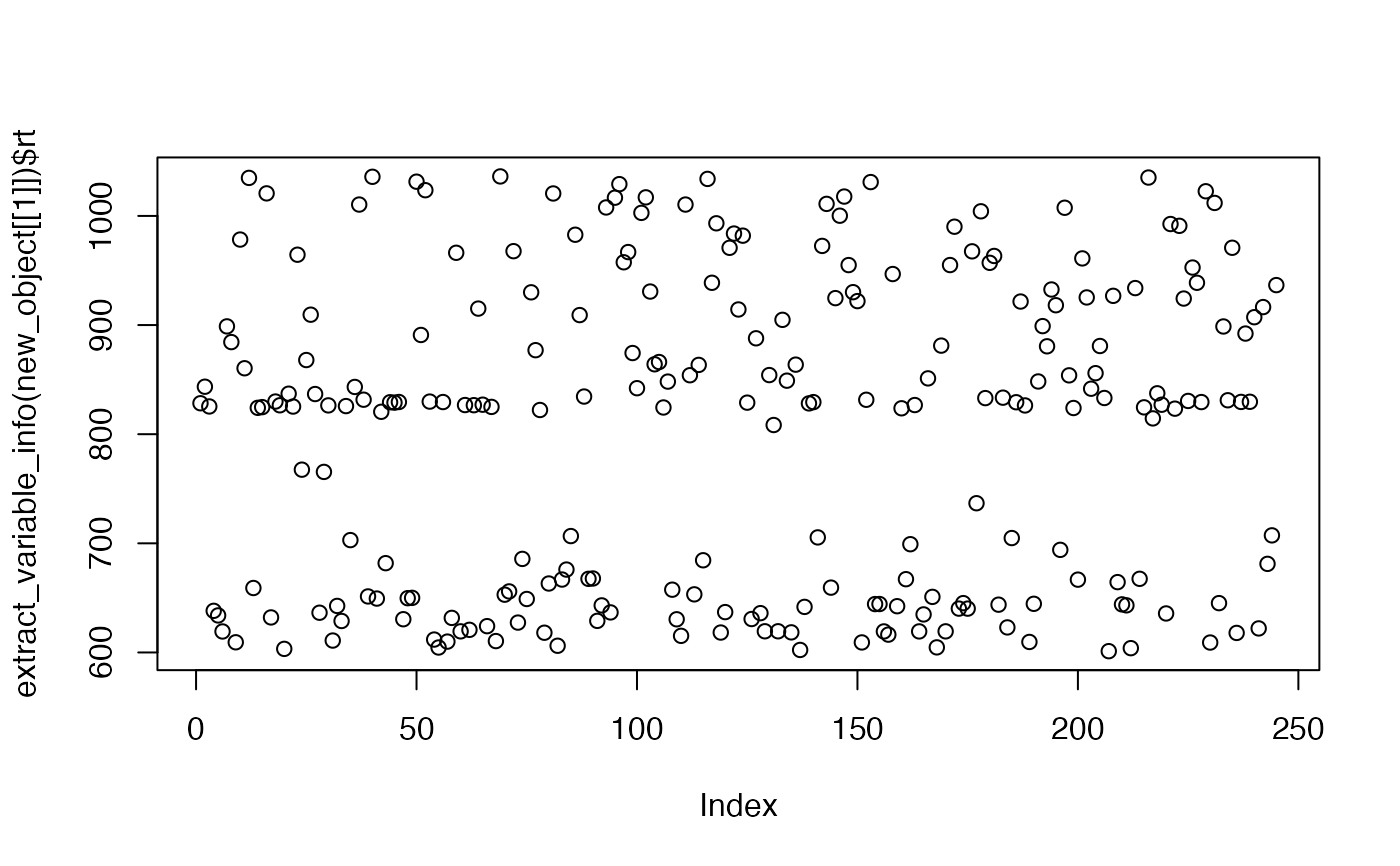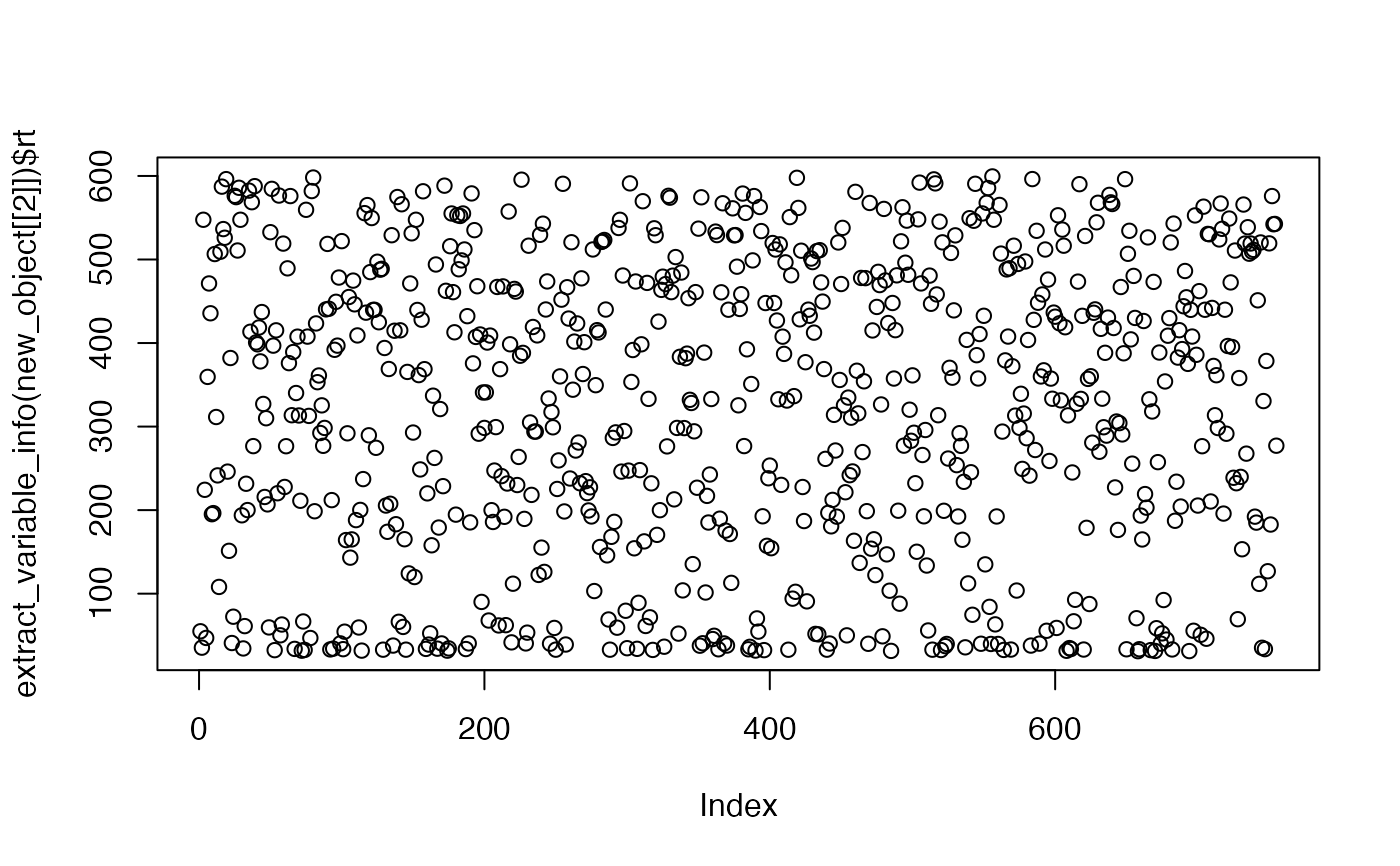Split one mass_dataset into several mass_dataset objects.

split_mass_dataset(object, by, fun)

## Arguments

object

(required) A mass_dataset class object.

by

column name of sample_info or variable_info

fun

function used to split by. For example, mz, should set it as > 100.

## Value

A list of mass_dataset.

## Author

Xiaotao Shen shenxt1990@outlook.com

## Examples

data("expression_data")
data("sample_info")
data("variable_info")

object =
create_mass_dataset(
expression_data = expression_data,
sample_info = sample_info,
variable_info = variable_info,
)

object <-
activate_mass_dataset(object, what = "sample_info")

new_object <-
split_mass_dataset(object = object, by = "group")

new_object %>% lapply(dim)
#> $Blank #> variables samples #> 1000 2 #> #>$QC
#> variables   samples
#>      1000         2
#>
#> $Subject #> variables samples #> 1000 4 #> new_object %>% lapply(colnames) #>$Blank
#>  "Blank_3" "Blank_4"
#>
#> $QC #>  "QC_1" "QC_2" #> #>$Subject
#>  "PS4P1" "PS4P2" "PS4P3" "PS4P4"
#>

object <-
activate_mass_dataset(object, what = "variable_info")

new_object <-
split_mass_dataset(object = object, by = "rt", fun = function(rt) rt > 600)

new_object %>% lapply(dim)
#> []
#> variables   samples
#>       245         8
#>
#> []
#> variables   samples
#>       755         8
#>
plot(extract_variable_info(new_object[])$rt)plot(extract_variable_info(new_object[])$rt)